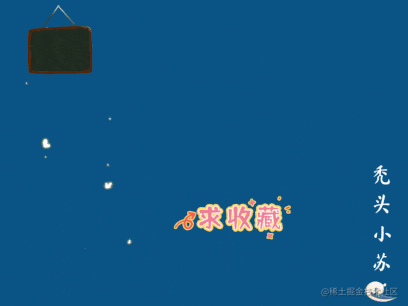# CV攻城獅入門VIT(vision transformer)之旅——VIT程式碼實戰篇

## theme: fancy

🍊作者簡介：禿頭小蘇，致力於用最通俗的語言描述問題

🍊近期目標：寫好專欄的每一篇文章

🍊支援小蘇：點贊👍🏼、收藏⭐、留言📩

# CV攻城獅入門VIT(vision transformer)之旅——VIT程式碼實戰篇

## 寫在前面

​  在上一篇，我們已經介紹了VIT的原理，是不是發現還挺簡單的呢！對VIT原理不清楚的請點選☞☞☞瞭解詳細。🌿🌿🌿那麼這篇我將帶大家一起來看看VIT的程式碼，主要為大家介紹VIT模型的搭建過程，也會簡要的說說訓練過程。

​  這篇VIT的模型是用於物體分類的，我們選擇的例子是花的五分類問題。關於花的分類，我之前也有詳細的介紹，是用卷積神經網路實現的，不清楚可以點選下列連結瞭解詳情：

​  程式碼部分依舊參考的是B站霹靂吧啦Wz視訊 ，強烈推薦大家觀看喔，你一定會收穫滿滿！！！🌾🌾🌾如果你看視訊中有什麼不理解的，可以來這篇文章尋找尋找答案喔。🌼🌼🌼

​  程式碼點選☞☞☞獲取。🥝🥝🥝

## VIT模型構建

​  這部分我以VIT-Base模型為例為大家講解，此模型的相關引數如下：

| Model | Patch size | Layers | Hidden Size | MLP size | Heads | Params | | :------: | :--------: | :----: | :---------: | :------: | :---: | :----: | | VIT-Base | 16*16 | 12 | 768 | 3072 | 12 | 86M |

​  在上程式碼之前，我們有必要了解整個VIT模型的結構。關於這點我在上一篇VIT原理詳解篇已經為大家介紹過，但上篇模型結構上的一些細節，像Droupout層，Encoder結構等等都是沒有體現的，這些只有閱讀原始碼才知道。下面給出整個VIT-Base模型的詳細結構，如下圖所示：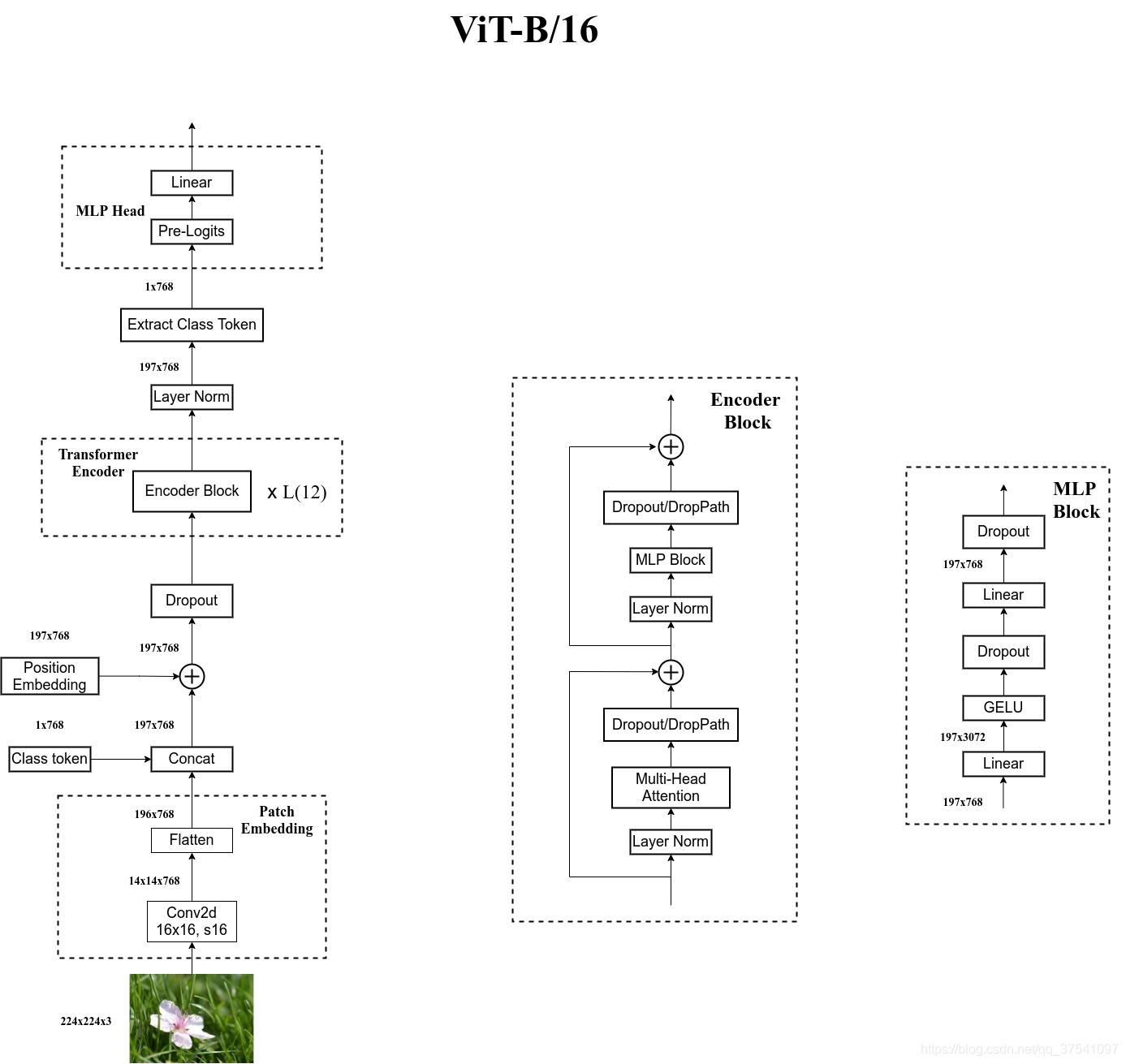​              圖片來自於霹靂吧啦Wz部落格

​  我們的程式碼是完全按照上圖結構搭建的，但在解讀程式碼之前我覺得很有必要再向大家強調一件事——你看我上文推薦的視訊或看我的程式碼解讀都只起到一個輔助的作用，你很難說光靠看就能把這些理解透徹。我當時看視訊的時候甚至很難完整的看完一遍，更多的還是靠自己一步一步的除錯來看每個操作後維度的變換。

​  我猜測可能有些同學還不是很清楚怎麼在`vit_model.py`進行除錯，其實很簡單，只需要建立一個全1的tensor來模擬圖片，將其當作輸入輸入網路即可，即可在`vit_model.py`檔案末尾加上下列程式碼：

```python if __name__ == '__main__': input = torch.ones(1, 3, 224, 224) # 1為batch_size (3 224 224)即表示輸入圖片尺寸 print(input.shape) model = vit_base_patch16_224_in21k() #使用VIT_Base模型,在imageNet21k上進行預訓練 output = model(input) print(output.shape)```

​  那麼下面我們就一步步的對程式碼進行解讀，首先我們先對輸入進行Patch_embedding操作，這部分我在理論詳解篇有詳細的介紹過，其就是採用一個卷積核大小為16*16，步長為16的卷積和一個展平操作實現的，相關程式碼如下：

```python class PatchEmbed(nn.Module): """ 2D Image to Patch Embedding """ def init(self, img_size=224, patch_size=16, in_c=3, embed_dim=768, norm_layer=None): super().init() img_size = (img_size, img_size) patch_size = (patch_size, patch_size) self.img_size = img_size self.patch_size = patch_size self.grid_size = (img_size // patch_size, img_size // patch_size) self.num_patches = self.grid_size * self.grid_size

``````    self.proj = nn.Conv2d(in_c, embed_dim, kernel_size=patch_size, stride=patch_size)
self.norm = norm_layer(embed_dim) if norm_layer else nn.Identity()

def forward(self, x):
B, C, H, W = x.shape
assert H == self.img_size and W == self.img_size, \
f"Input image size ({H}*{W}) doesn't match model ({self.img_size}*{self.img_size})."

# flatten: [B, C, H, W] -> [B, C, HW]
# transpose: [B, C, HW] -> [B, HW, C]
x = self.proj(x).flatten(2).transpose(1, 2)
x = self.norm(x)
return x
``````

```

​  其實我覺得我再怎麼解釋這個程式碼的效果都不會很好，你只要在這裡打上一個斷點，這個過程就一目瞭然了。所以這篇文章可能就更傾向於讓大家熟悉一下整個模型搭建的過程，具體細節大家可自行除錯！！！🌻🌻🌻

​  這步結束後，你會發現現在x的維度為(1，196，768)。其中1為batch_size數目，我們之前將其設為1。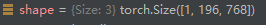​  接著我們會將此時的x和`Class token`拼接，相關程式碼如下：

```python

# 定義一個可學習的Class token

self.cls_token = nn.Parameter(torch.zeros(1, 1, embed_dim)) # 第一個1為batch_size embed_dim=768 cls_token = self.cls_token.expand(x.shape, -1, -1) # 保證cls_token的batch維度和x一致 if self.dist_token is None: x = torch.cat((cls_token, x), dim=1) # [B, 197, 768] self.dist_token為None,會執行這句 else: x = torch.cat((cls_token, self.dist_token.expand(x.shape, -1, -1), x), dim=1)

```

​  同樣可以來看看拼接後的維度，如下圖：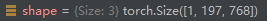​  繼續進行下一步——位置編碼。位置編碼是和上步得到的x進行相加的操作，相關程式碼如下:

```python # 定義一個可學習的位置編碼 self.pos_embed = nn.Parameter(torch.zeros(1, num_patches + self.num_tokens, embed_dim)) #這個維度為（1，197，768） x = x + self.pos_embed```

​  經過位置編碼輸入的維度並不會發生變換，如下：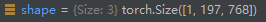​  位置編碼過後，還會經過一個Dropout層，這並不會改變輸入維度，相信大家對這個就很熟悉了，就不過多介紹了。

```python class Block(nn.Module): def init(self, dim, num_heads, mlp_ratio=4., qkv_bias=False, qk_scale=None, drop_ratio=0., attn_drop_ratio=0., drop_path_ratio=0., act_layer=nn.GELU, norm_layer=nn.LayerNorm): super(Block, self).init() self.norm1 = norm_layer(dim) self.attn = Attention(dim, num_heads=num_heads, qkv_bias=qkv_bias, qk_scale=qk_scale, attn_drop_ratio=attn_drop_ratio, proj_drop_ratio=drop_ratio) # NOTE: drop path for stochastic depth, we shall see if this is better than dropout here self.drop_path = DropPath(drop_path_ratio) if drop_path_ratio > 0. else nn.Identity() self.norm2 = norm_layer(dim) mlp_hidden_dim = int(dim * mlp_ratio) self.mlp = Mlp(in_features=dim, hidden_features=mlp_hidden_dim, act_layer=act_layer, drop=drop_ratio)

``````def forward(self, x):
x = x + self.drop_path(self.attn(self.norm1(x)))   #🌰🌰🌰上文描述的在這喔🌰🌰🌰
x = x + self.drop_path(self.mlp(self.norm2(x)))    #這是encode結構的後半部分
return x
``````

```

```python class Attention(nn.Module): def init(self, dim, # 輸入token的dim num_heads=8, qkv_bias=False, qk_scale=None, attn_drop_ratio=0., proj_drop_ratio=0.): super(Attention, self).init() self.num_heads = num_heads head_dim = dim // num_heads self.scale = qk_scale or head_dim ** -0.5 self.qkv = nn.Linear(dim, dim * 3, bias=qkv_bias) self.attn_drop = nn.Dropout(attn_drop_ratio) self.proj = nn.Linear(dim, dim) self.proj_drop = nn.Dropout(proj_drop_ratio)

``````def forward(self, x):
# [batch_size, num_patches + 1, total_embed_dim]
B, N, C = x.shape

# qkv(): -> [batch_size, num_patches + 1, 3 * total_embed_dim]
qkv = self.qkv(x).reshape(B, N, 3, self.num_heads, C // self.num_heads).permute(2, 0, 3, 1, 4)
q, k, v = qkv, qkv, qkv  # make torchscript happy (cannot use tensor as tuple)

# @: multiply -> [batch_size, num_heads, num_patches + 1, num_patches + 1]
attn = (q @ k.transpose(-2, -1)) * self.scale
attn = attn.softmax(dim=-1)
attn = self.attn_drop(attn)

# reshape: -> [batch_size, num_patches + 1, total_embed_dim]
x = (attn @ v).transpose(1, 2).reshape(B, N, C)
x = self.proj(x)
x = self.proj_drop(x)
return x
``````

```

​  光看確實難以發現其中的很多細節，那就盡情的除錯吧！！！🌼🌼🌼這部分也不會改變x的尺寸，如下：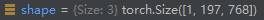​  下一步同樣是一個Layer Normalization層，接著是MLP Block，最後是一個Droppath加一個殘差連結。這一部分還值得說的就是這個MLP Bolck了，但其實也非常簡單，主要就是兩個全連線層，相關程式碼如下：

```python class Mlp(nn.Module): """ MLP as used in Vision Transformer, MLP-Mixer and related networks """ def init(self, in_features, hidden_features=None, out_features=None, act_layer=nn.GELU, drop=0.): super().init() out_features = out_features or in_features hidden_features = hidden_features or in_features self.fc1 = nn.Linear(in_features, hidden_features) self.act = act_layer() self.fc2 = nn.Linear(hidden_features, out_features) self.drop = nn.Dropout(drop)

``````def forward(self, x):
x = self.fc1(x)
x = self.act(x)
x = self.drop(x)
x = self.fc2(x)
x = self.drop(x)
return x
``````

```

​  需要提醒大家的是上述程式碼的hidden_features其實就是一開始模型引數中MLP size，即3072。

​  這樣一個encoder Block就介紹完了，接著只需要重複這個Block 12次即可。這部分相關程式碼如下：

```python self.blocks = nn.Sequential(*[ Block(dim=embed_dim, num_heads=num_heads, mlp_ratio=mlp_ratio, qkv_bias=qkv_bias, qk_scale=qk_scale, drop_ratio=drop_ratio, attn_drop_ratio=attn_drop_ratio, drop_path_ratio=dpr[i], norm_layer=norm_layer, act_layer=act_layer) for i in range(depth) ])

x = self.blocks(x) ```

​  注意輸入輸出這個encoder Block前後，x的維度同樣沒有發生變化，仍為（1，197，768）。接著會進行Layer Normalization操作。然後要通過切片的方式提取出Class Token，程式碼如下：

```python if self.dist_token is None: return self.pre_logits(x[:, 0]) #self.dist_token=None 執行此句 else: return x[:, 0], x[:, 1]```

​  你會發現上述程式碼中會存在一個pre_logits()函式，這個函式其實就是一個全連線層加上一個Tanh啟用函式，如下：

```python

# Representation layer

if representation_size and not distilled: self.has_logits = True self.num_features = representation_size self.pre_logits = nn.Sequential(OrderedDict([ ("fc", nn.Linear(embed_dim, representation_size)), ("act", nn.Tanh()) ])) else: self.has_logits = False self.pre_logits = nn.Identity() ```

​  可以發現，這部分不是總存在的。當representation_size=None時，此部分只是一個恆等對映，即什麼都不做。關於representation_size何時取何值，我這裡做一個簡要的說明。當我們的預訓練資料集是ImageNet時，representation_size=None，即此時什麼都不做；當預訓練資料集為ImageNet-21k時，representation_size是一個特定的值，至於是多少是不定的，這和是Base、Large或Huge模型有關，我們這裡以Base模型為例，representation_size=768。

​  經過pre_logits後，還有最後一個全連線層用於最終的分類。相關程式碼如下：

```python self.head = nn.Linear(self.num_features, num_classes) if num_classes > 0 else nn.Identity() x = self.head(x)```

​  到這裡，VIT模型的搭建就全部介紹完啦，看到這裡的話，為自己鼓個掌吧👏👏👏

## VIT 訓練指令碼

​  VIT訓練部分和之前我用神經網路搭建的花類識別訓練指令碼基本是一樣的，不清楚的可以先去看看之前的文章。這裡我給大家講講怎麼進行訓練。其實你需要修改的地方只有兩處，第一是資料集的路徑，在程式碼中設定預設路徑如下：

```python parser.add_argument('--data-path', type=str, default="/data/flower_photos")```

​  我們只需要將`"/data/flower_photos"`修改成我們對應的資料集路徑即可。需要注意的是這裡路徑要指定到flower_photos資料夾，否則檢測不到圖片，這裡和之前講的還是有點差別的。

​ 還有一處你需要修改的地方為預訓練權重的位置，程式碼中預設路徑如下：

```python

# 預訓練權重路徑，如果不想載入就設定為空字元

parser.add_argument('--weights', type=str, default='./vit_base_patch16_224_in21k.pth', help='initial weights path') ```

​  我們需要將`'./vit_base_patch16_224_in21k.pth'`換成自己下載預訓練權重的地址。需要注意的時這裡的預訓練權重需要和你建立模型時選擇的模型是一樣的，即你選擇了VIT_Base模型並在ImageNet21k上做預訓練，你就要使用`./vit_base_patch16_224_in21k.pth`的預訓練權重。

​  最後我們訓練的權重會儲存在當前資料夾下的weights資料夾下，沒有這個資料夾會建立一個新的，相關程式碼如下：

```python torch.save(model.state_dict(), "./weights/model-{}.pth".format(epoch))```

## VIT分類任務實驗結果

​  這裡我們來看看花的五分類訓練結果：

`不使用預訓練模型訓練10輪：`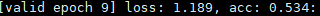`不使用預訓練權重訓練50輪：`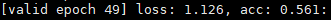`使用預訓練權重訓練10輪：`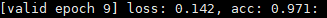​  通過上面的三個實驗你可以發現，VIT模型不使用預訓練權重進行訓練的話效果是非常差的，我們用ResNet網路不使用預訓練權重訓練50輪大概能達到0.79左右的準確率，而ViT只能達到0.561；但是使用了預訓練模型的ResNet達到了0.915，而VIT高達0.971，效果是非常不錯的。所以VIT是非常依賴預訓練的，且預訓練資料集越大，效果往往越好。🥂🥂🥂

​  最後我們來看看預測部分，下圖為檢測鬱金香的概率：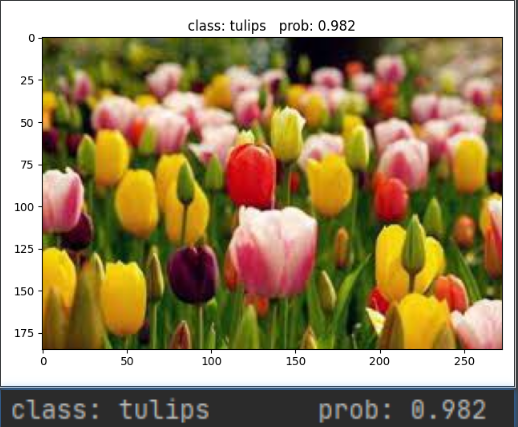## 小結

​  到這裡，VIT程式碼實戰篇就介紹完了。同時CV攻城獅入門VIT(vision transformer)之旅的三篇文章到這裡也就告一個段落了，希望大家能夠有所收穫吧！！！🌾🌾🌾

​  這裡預告一下，後期我打算出Swin Transformer的教程，這個模型才是目前真正霸榜的存在，敬請期待吧！！！🥗🥗🥗